### Home > CALC > Chapter 4 > Lesson 4.3.1 > Problem4-98

4-98.
1. Examine the following integrals. Consider the multiple tools available for evaluating integrals and use the best strategy for each. After evaluating the integral, write a short description of your method. Homework Help ✎

1.2.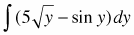3.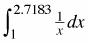4.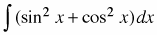5.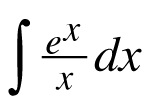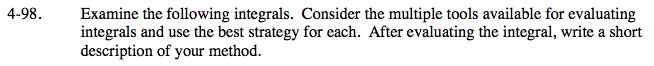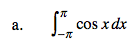To make this problem quicker to solve, remember that
y = cosx is an even function.

$=2\int_{0}^{\pi}\text{cos}xdx=2\left ( \text{sin}x\left|\begin{matrix} \pi \\ 0 \end{matrix}\right. \right )=2[\text{sin}\pi -\text{sin}0]=0$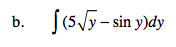Notice that this is an indefinite integral. Don't forget the +C.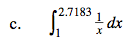2.7183 ≈ e (Euler's number) and the derivative of ln(x) is x−1.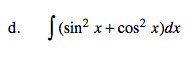Think! Trig identity. Simplify the integrand before you integrate.